Test: Power Systems- 7

# Test: Power Systems- 7

Test Description

## 10 Questions MCQ Test GATE Electrical Engineering (EE) 2023 Mock Test Series | Test: Power Systems- 7

Test: Power Systems- 7 for Electrical Engineering (EE) 2023 is part of GATE Electrical Engineering (EE) 2023 Mock Test Series preparation. The Test: Power Systems- 7 questions and answers have been prepared according to the Electrical Engineering (EE) exam syllabus.The Test: Power Systems- 7 MCQs are made for Electrical Engineering (EE) 2023 Exam. Find important definitions, questions, notes, meanings, examples, exercises, MCQs and online tests for Test: Power Systems- 7 below.
Solutions of Test: Power Systems- 7 questions in English are available as part of our GATE Electrical Engineering (EE) 2023 Mock Test Series for Electrical Engineering (EE) & Test: Power Systems- 7 solutions in Hindi for GATE Electrical Engineering (EE) 2023 Mock Test Series course. Download more important topics, notes, lectures and mock test series for Electrical Engineering (EE) Exam by signing up for free. Attempt Test: Power Systems- 7 | 10 questions in 30 minutes | Mock test for Electrical Engineering (EE) preparation | Free important questions MCQ to study GATE Electrical Engineering (EE) 2023 Mock Test Series for Electrical Engineering (EE) Exam | Download free PDF with solutions
 1 Crore+ students have signed up on EduRev. Have you?
Test: Power Systems- 7 - Question 1

### Determine the fault current in a system following a double line to ground short circuit at the terminals of a star connected synchronous generator operating initially on an open circuit voltage of 1.0 pu. The positive, negative and zero sequences reactance of the generator are, respectively j 0.35, j 0.25 and j 0.20 and the star point is isolated from ground.

Detailed Solution for Test: Power Systems- 7 - Question 1

Since the star point is isolated from ground LLG fault is just like LL fault.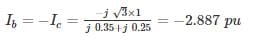*Answer can only contain numeric values
Test: Power Systems- 7 - Question 2

### A 50 Hz overhead Line has Line to each capacitance of 2 μF. It is decided to use an earth fault neutralizer. The reactance to neutralizer. The reactance to neutralize the capacitance of 80% of the length of the line is – (in ohms)

Detailed Solution for Test: Power Systems- 7 - Question 2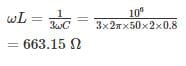Test: Power Systems- 7 - Question 3

### A positive, negative and zero sequence impedance of 3ϕ generator are Z1, Z2 and Z0 respectively. For a line to line fault with fault impedance ZF, the fault current is IF1 = KIF. Where IF is the fault current with zero fault impedance. The relation between ZF and K is

Detailed Solution for Test: Power Systems- 7 - Question 3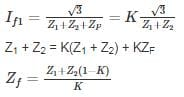*Answer can only contain numeric values
Test: Power Systems- 7 - Question 4

A three phase 15 MVA, 13 kV alternator has an initial reactance of 5%. The series reactor needed to restrict the short circuit current of the alternator to 10 times of full load current is _____ (in Ω).

Detailed Solution for Test: Power Systems- 7 - Question 4

Short circuit current ISC =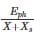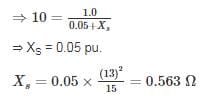Test: Power Systems- 7 - Question 5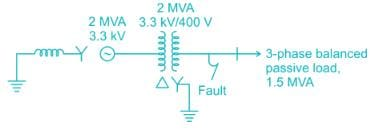A 2 MVA, 3.3 kV, three-phase generator as the following reactance’s on its own base:

+ve sequence reactance, x1 = j0.10 p.u.

-ve sequence reactance, x2 = j0.10 p.u.

Zero sequence reactance, x0 = j0.05 p.u.

Reactance from neutral to ground, xn = j0.05 p.u.

The generator supplies a 3-phase, 1.5 MVA balanced passive load through a 3-phase, 2.0 MVA, 3.3 kV/400 V, delta-star transform with leakage reactance of 10%, as shown in the figure. A single-line-to-ground fault takes place at the low voltage side of the transformer. The current flowing from the transformer to the fault is (Assume base MVA is 1.5 MVA)

Detailed Solution for Test: Power Systems- 7 - Question 5

The generator supplies a 3-phase, 1.5 MVA balanced passive load.

so, the system works under a base load of 1.5 MVA

The generator reactance on a base load of 1.5 MVA are as follows.
+ve sequence reactance x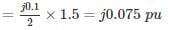-ve sequence reactance x=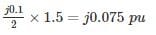zero sequence reactance x0 =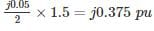Reactance form neutral to ground xn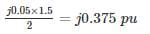leakage reactance of transformer x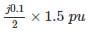= j0.075 pu

Positive sequence network for the above system is,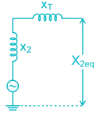X1eq = x+ xT

= j0.075 + j0.075

= j0.15 pu

Negative sequence network for above system is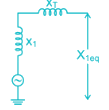X2eq = x2 + xT = j0.075 + j0.075

= j0.15 pu

Zero sequence network for above system is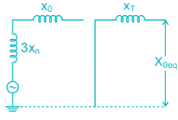X0eq = xT = j 0.075 pu

For a line to ground fault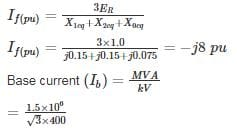= 2165.06 A

Fault current (If) = (Ib) × (If(pu))

= 2165.06 × 8

= 17320.5 A

*Answer can only contain numeric values
Test: Power Systems- 7 - Question 6

A 10 MVA, 6.6 kV generator has percentage reactances to positive, negative and zero sequence currents of 15%, 10%, and 5% respectively and it's neutral is solidly grounded. The generator is unloaded and excited to its rated voltage. The fault current (in kA) will be when a single line to ground fault develops on phase a of the generator. The phase sequence is ABC.

Detailed Solution for Test: Power Systems- 7 - Question 6

On base MVA of 10 and base voltage of 6.6 kV

Z1 = j 0.15 pu

Z2 j 0.10 pu

Z0 = j 0.05 pu

Zn = 0, Zf = 0

For L-G fault on phase ‘a’

Ib = Ic = 0 and Va = 0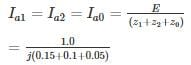= -j 3.33 pu

Fault current, If = Ia = 3 Ia1 = -j 10 pu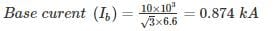Fault current = Ib × If (pu)

= 8.74 kA

*Answer can only contain numeric values
Test: Power Systems- 7 - Question 7

For an unloaded generator, at rated voltage, the magnitude of fault current for a three-phase fault is the same as LG fault. The reactances of the generator are

x1 = 0.2 pu, x2 = 0.15 pu, x0 = 0.05 pu

The value of the neutral grounding reactance is ______ (in pu)

Detailed Solution for Test: Power Systems- 7 - Question 7

x1 = 0.2 pu, x2 = 0.15 pu, x0 = 0.05 pu

Magnitude of fault current for three phase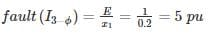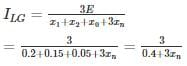Given that, I3-ϕ­ = ILG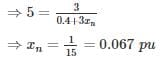Test: Power Systems- 7 - Question 8

The severity of line to ground and three phase faults is to be same. If the terminal voltage is 1.0 pu, Z1 = Z2 = j 0.1 pu and Z0 = j 0.05 pu for an alternator, then the required inductive reactance for neutral grounding is

Detailed Solution for Test: Power Systems- 7 - Question 8

Given that,

Z1 = Z2 = j 0.1 pu

Z0 = j 0.05 pu

Ea = 1 pu

For line to ground fault most server current = In = 3 Iao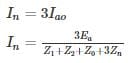For 3 - phase fault, fault current =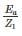As per the equation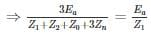⇒ 3z1 = Z1 + Z2 + Z0 + 3Zn

⇒ 3Zn = 2Z1 - Z2 - Z0

⇒ 3Zn = j2(0.1) – j0.1 – j 0.05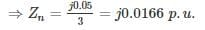*Answer can only contain numeric values
Test: Power Systems- 7 - Question 9

The 33 kV busbars of a station are in two sections A and B separated by a reactor. Section A is fed from four 10 MVA generators each having a reactance of 20% section B is fed from the grid through a 50 MVA transformer of 10% reactance. The circuit breakers have each a rupturing capacity of 500 MVA. Find the reactance (in Ω) of the reactor to prevent the circuit breakers from being overloaded, if a symmetrical three-phase fault occurs on an outgoing feeder connected for it. (Take base voltage of 33 KV and base MVA of 50).

Detailed Solution for Test: Power Systems- 7 - Question 9

The one-line diagram of the system is shown in figure. Given that base voltage = 33 kV

base MVA = 50 MVA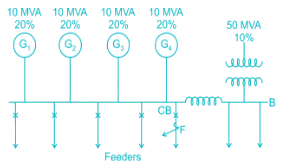Per unit reactance of generators and transformer are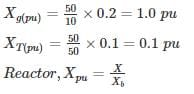For a symmetrical three-phase fault at point F on an outgoing feeder connected to A, the reactance diagram with voltage sources short circuited will be as shown below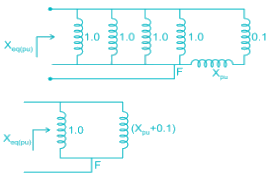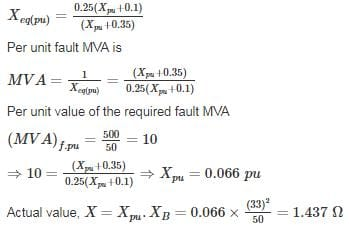Test: Power Systems- 7 - Question 10

Three 6.6 kV generators A, B and C each of 10% leakage reactance and MVA ratings 40, 50 and 25, respectively are interconnected electrically as shown in the figure, by a tie bar through current limiting reactors, each of 12% reactance based upon the rating of the machine to which it is connected. A three-phase feeder is supplied from the bus bar of generator A at a line voltage of 6.6 kV. The feeder has a resistance of 0.06 Ω/phase and an indicative reactance of 0.12 Ω/phase. Estimate the maximum MVA that can be fed into asymmetrical short circuit at the far end of the feeder.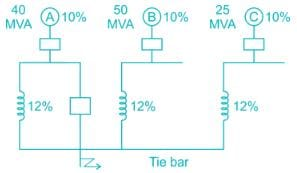Detailed Solution for Test: Power Systems- 7 - Question 10

Base = 50 MVA, 6.6 kV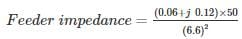= (0.069 + j 0.138) pu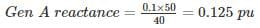Gen B reactance = 0.12 pu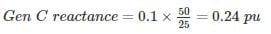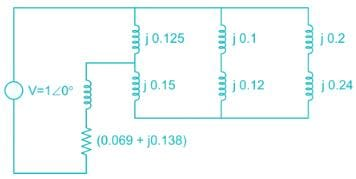Zeq = 0.069 + j 0.138 + j 0.125 [(j 0.15) + (j 0.22 || j 0.44)]

= 0.069 + j 0.226 = 0.236 ∠73°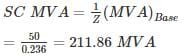## GATE Electrical Engineering (EE) 2023 Mock Test Series

22 docs|274 tests
Information about Test: Power Systems- 7 Page
In this test you can find the Exam questions for Test: Power Systems- 7 solved & explained in the simplest way possible. Besides giving Questions and answers for Test: Power Systems- 7, EduRev gives you an ample number of Online tests for practice

## GATE Electrical Engineering (EE) 2023 Mock Test Series

22 docs|274 tests(Scan QR code)